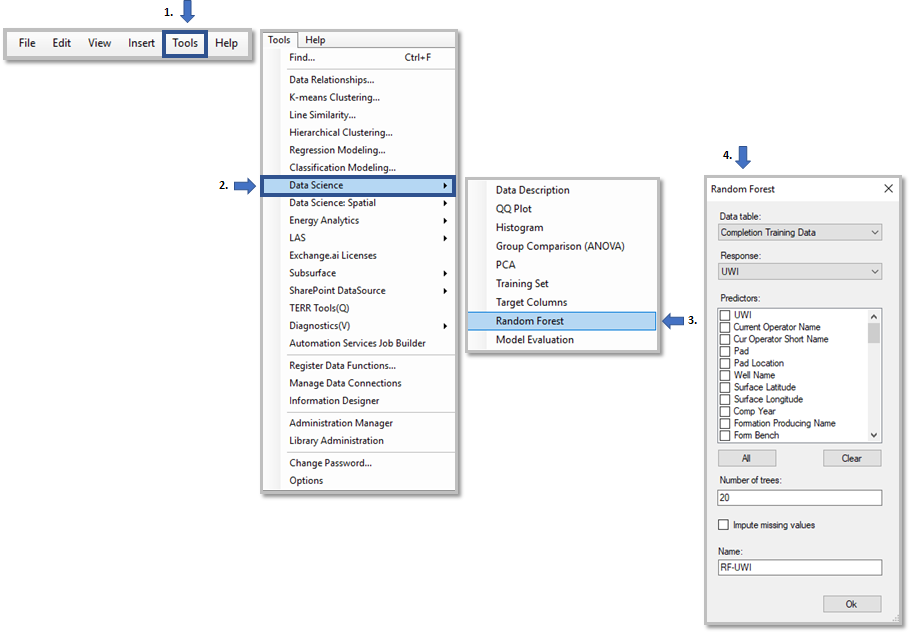### Purpose

The Random Forest model is a form of multivariate analysis.  It uses an ensemble learning method for classification and regression.  The model constructs a "forest" of decision trees and generally does not overfit.

#### Limitations:

• If a column has been selected for the model, it cannot have nulls (unless you impute)
• Does not work with the Analytics Model panel in Spotfire

#### Prep:

• First run data description to understand underlying data distributions
• Investigate collinearity of input variables using PCA
• Data that you want to predict on should be appended to the input data set, but have missing values in the response column
• This technique can be used to make a hold-out set for validation

#### Steps to Run:

1. Go to the Tools menu
2. Select the Data Science option
3. Select the Random Forest option
4. Enter the data table, response column, predictor columns, number of trees, imputation choice and name.#### Random Forest Inputs

1. Data table
2. Response column (dependent variable)
3. Predictor columns (independent variables used to predict dependent variables)
4. Number of trees
5. Checkbox to impute missing values (yes/no)
6. Name#### Outputs:

• There are 6 outputs.
• The OOB Error Rate - Number of Trees line chart shows the reduction in error as more trees are added to the ensemble
• The Definitions text area provides an explanation of the error rate and % variance.
• The Training Set Stats table shows a print out of summary information on the model parameters and goodness of fit
• The Importance per Variable bar chart shows which variables in the model were the most important.
• The Actual vs Predicted scatter plot shows the values of the predicted response variable versus the actual values of the response variable in the data set.
• The predicted response vs predictor column scatter plot shows how closely the model predicted the response column based on the unique values of all the variables in the model.

Note: The new prediction data will be added to a column in the original data table

Try using other visualization and color by the results predicted.
Example:#### Interpretation

• In the Actuals vs Predicted scatter plot, the closer the markers are to the straight line fit, the more accurate the model.
Variable importance provides a relative ranking of the variables

#### How to filter a subset of data

1. Open the filter panel by clicking the filter icon on the top bar.
2. Choose the correct filtering scheme
3. Click " Refresh data table" on visualization.
• This syncs with all the other visualization on Random Forest.See RAI Random Forest video below
For additional information on RAI Data Science Toolkit documentation, click here.